Vol. 43
Latest Volume
All Volumes
All Issues
PIER
Vol. 43, 305-333, 2003
Scattering by Parallel Cylindrical Posts with Conducting Strips
The theory of scattering in open and closed areas by a novel structure of a dielectric-metallic post is developed with the use of a combination of a modified iterative scattering procedure and an orthogonal expansion method. The scattered field pattern for open structures and frequency responses of the transmission and re ection coefficients in a rectangular waveguide are derived. The turn and displacement of the post in a waveguide allows to change the resonance frequency of the circuit. A good agreement is obtained between the results of our method and those received from FDTD simulations and measurements. The proposed model is much faster than the FDTD method used for comparison. The computation time is independent of the kind of dielectric material the post is made of.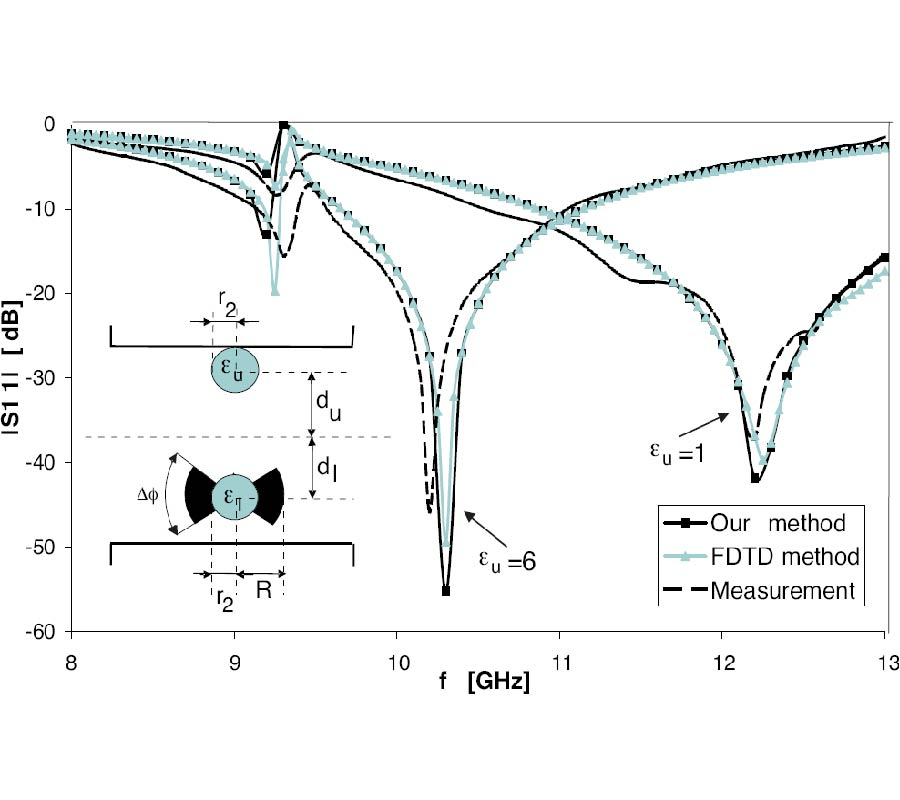PIER
Vol. 43, 287-304, 2003
Genetically Evolved Phase-Aggregation Technique for Linear Arrays Control
This paper presents a modified Genetic Algorithm (GA) technique in which the large phase perturbations are calculated by aggregating small phase increments. The proposed aggregation GA technique overcomes the major drawback of the large solution space required by the classical GA techniques. The proposed method adopts small ranges for increments of the parameters and the optimality is reached via aggregation of the best increments of phases. Consequently, the GA searches in a smaller solution space and finds the solution with reduced number of iterations. Simulation results show the achieved improvement of the proposed technique over the classical GA. The suppressed sectors using phase-only control are accomplished with and without element failures. Problems like imposing symmetrical nulls around the mainbeam and compensation for the failure of center element have been achieved.PIER
Vol. 43, 273-285, 2003
Modeling of RF Absorber for Application in the Design of Anechoic Chamber
A proper model of RF absorber must be developed based on information such as absorber reflectivity, in magnitude and phase, for various angles of incidence, and for parallel and perpendicular polarizations. Unfortunately, these data are not available due to the practical limitations of the test fixtures to measure the RF absorber performance. Manufacturer data sheets normally specify only the magnitude of the absorber reflectivity for normal incidence. A model has been developed in this paper for pyramidal RF absorber with pyramid length shorter than a quarter wavelength and poor reflectivity performance. Since the reflection from the metal backing would be much higher than the reflection and scattering from the pyramid tips, the metal boundary may be modeled as a lossy dielectric with certain effective dielectric constant, εeff , and effective conductivity, Ïƒeff , and the thickness extends to infinity. The appropriate values of εeff and Ïƒeff can be derived based on the reflectivity information given by the manufacturer's data sheet. The reflectivity at oblique incidence is calculated and compared with the results of method of homogenization and moment method. A reasonable match between the different models is obtained. The plane-boundary dielectric model can be used to evaluate the degradation of reflectivity level with respect to angle of incidence. It can be used in a simulation tool for design of anechoic chamber.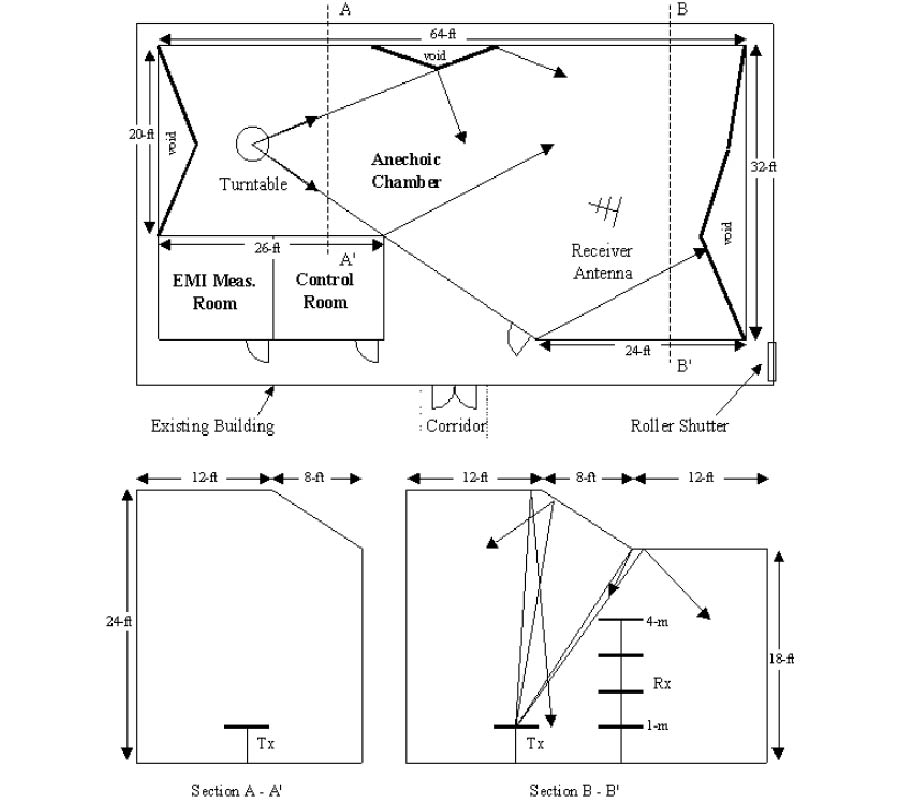PIER
Vol. 43, 257-271, 2003
An Investigation of Magnetic Antennas for Ground Penetrating Radar
For ground penetrating radar (GPR), smaller antennas would provide considerable practical advantages. Some of which are: portability; ease of use; and higher spatial sampling. A theoretical comparison of the fundamental limits of a small electric field antenna and a small magnetic field antenna shows that the minimum Q constraints are identical. Furthermore, it is shown that only the small magnetic loop antenna can be constructed to approach, arbitrarily closely, the fundamental minimum Q limit. This is achieved with the addition of a high permeability material which reduces energy stored in the magnetic fields. This is of special interest to some GPR applications. For example, applications requiring synthetic aperture data collection would benefit from the increased spatial sampling offered by electrically smaller antennas. Low frequency applications may also benefit, in terms of reduced antenna dimensions, by the use of electrically small antennas. Under these circumstances, a magnetic type antenna should be considered in preference to the typical electric field antenna. Numerical modeling data supports this assertion.PIER
Vol. 43, 239-256, 2003
High-Impedance Surfaces Based on Self-Resonant Grids. Analytical Modelling and Numerical Simulations
A new variant of artificial high-impedance surfaces is suggested and studied. This is a thin composite layer consisting of a dielectric layer with a planar self-resonant grid from metal strips on its surface. Every grid element is connected to the ground plane with a metal pin. We use an analytical model which has been recently developed for a similar structure. The advantages of the new structure (decreasing the resonant frequency for fixed period and thickness,further angular stabilization of surface impedance for the TE-incidence of waves) are studied and explained. The analytical model is compared with numerical simulations. It predicts quite well the resonant frequencies of the artificial surface for different angles of incidence however is not enough accurate for calculating the values of the surface impedance.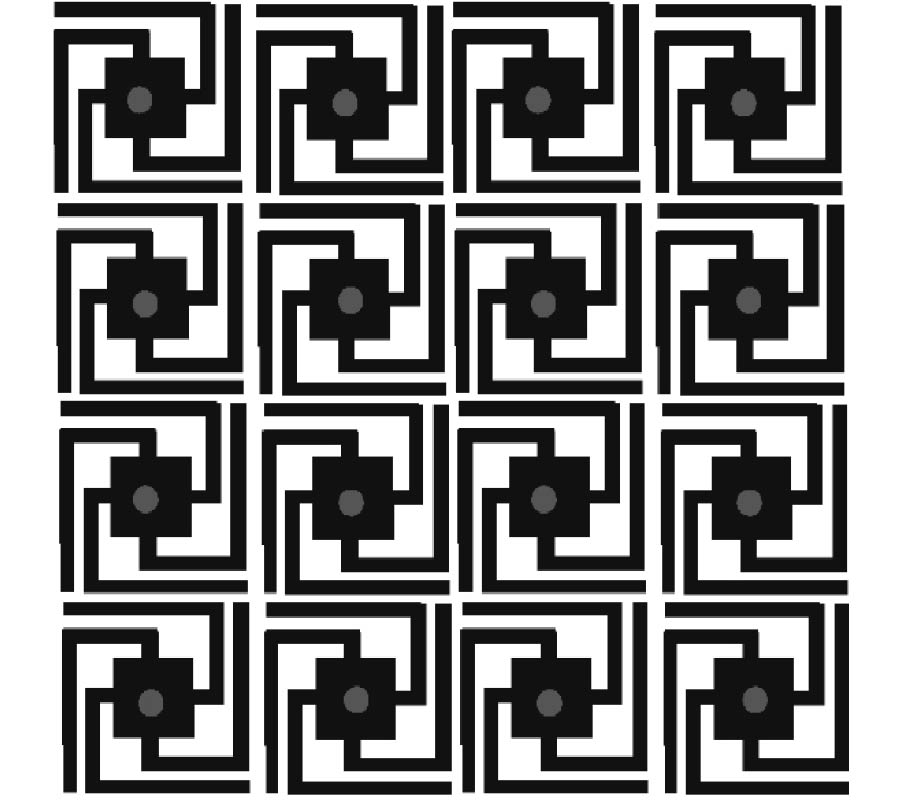PIER
Vol. 43, 181-237, 2003
Rigorous Coupled Wave Analysis of Bipolar Cylindrical Systems: Scattering from Inhomogeneous Dielectric Material, Eccentric, Composite Circular Cylinders
Rigorous Coupled Wave Analysis (RCWA) in bipolar coordinates for the first time is used to study electromagnetic (EM) scattering from eccentric, circular, multi-cylinder systems for which spatially, non uniform material (dielectric permittivity) occupies the regions between the interfaces of the cylinders. The bipolar RCWA algorithm presented herein consists of three basic steps which are; (1) solving Maxwell's equations in bipolar coordinates using a state variable (SV) formulation; (2) solving Maxwell's equations in the spatially uniform regions exterior to the inhomogeneous scattering object in terms of circular, cylindrical Bessel-Hankel functions; and (3) enforcement of EM boundary matching equations which leads to a final matrix equation solution of the system. In the paper extensive use of the residue theorem of complex variable theory was made in order to find fast and exact evaluations of the EM boundary interaction integrals that arose between the bipolar, SV solutions and the Hankel- Bessel solutions. In this paper very extensive reliance on the work of A. A. Kishk, R. P. Parrikar and A. Z. Elsherbeni  who studied EM scattering from uniform material multi-eccentric circular cylinders (called herein the KPE algorithm) was made in order to validate the numerical results of the bipolar RCWA algorithm. In this paper, two important system transfer matrices, called the Bessel transfer matrix (based on the KPE algorithm) and called the bipolar SV transfer matrix, were developed in order to validate the numerical accuracy of the RCWA algorithm. The Bessel and SV transfer matrices were very useful for validation purposes because, from the way they were both formulated, they could be meaningfully compared to one another, matrix element to matrix element. In the paper extensive numerical results are presented for EM scattering from spatially uniform and non uniform multi-eccentric, composite cylinder systems, including calculation of three dimensional plots of the electric and magnetic fields and including calculation of the back and bistatic scattering widths associated with the scattering systems. Also included are three tables of data documenting peak and RMS errors that occur between the KPE and RCWA algorithms when the number of modes are changed, the number of layers in the RCWA algorithm are varied and when the angle of incidence is varied.PIER
Vol. 43, 163-179, 2003
Topological Intensity Shifts of Electro-Magnetic Field in Lobachevskian Spaces. Olbers Paradox Solved, Deep Space Communication, and the New Electromagnetic Method of Gravitational Wave Detection
The major new result is the behavior of the intensity of electromagnetic radiationinLobac hevskian (hyperbolic) spaces. Equation (2) expresses change in intensity vs. space curvature and distance. Non existence of Olbers paradox in a Lobachevskian universe is shown. A new electromagnetic method for detection of gravitational waves is proposed. Explanation of observed perioditicy in redshifts is given. Problems of deep space communications are discussed.PIER
Vol. 43, 143-162, 2003
The Equivalence of Inclined Uniaxial and Biaxial Electrical Anisotropy in Inhomogeneous Two-Dimensional Media for Homogeneous TM-Type Plane Wave Propagation Problems
For a homogeneous TM-type wave propagating in a two-dimensional half space with both vertical and horizontal inhomogeneities, where the TM-type wave is aligned with one of the elements of the conductivity tensor, it is shown using exact solutions to boundary value problems that the shearing term in the homogeneous Helmholtz equation for inclined uniaxial anisotropic media unequivocally vanishes and solutions need only be sought to the homogeneous Helmholtz equation for fundamental biaxial anisotropic media. This implies that those problems posed with an inclined uniaxial conductivity tensor can be identically stated with a fundamental biaxial conductivity tensor, provided that the conductivity values are the reciprocal of the diagonal terms from the Euler rotated resistivity tensor. The applications of this for numerical methods of solving arbitrary two-dimensional problems for a homogeneous TM-type wave is that they need only to approximate the homogeneous Helmholtz equation and neglect the corresponding shearing term.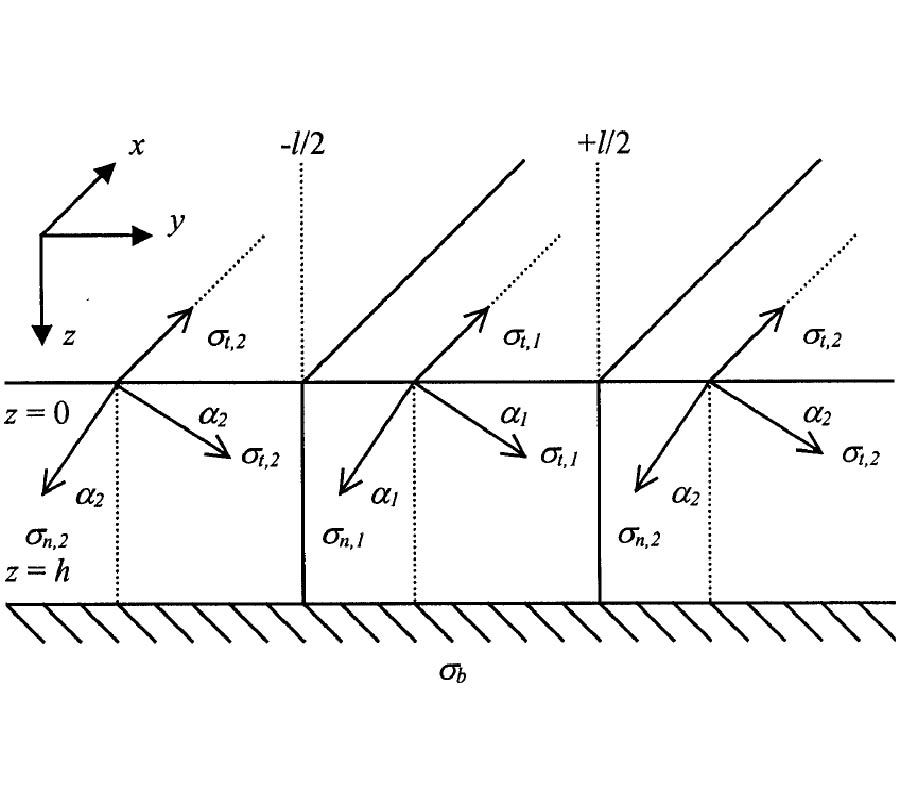PIER
Vol. 43, 123-142, 2003
2D Cavity Modeling Using Method of Moments and Iterative Solvers
The method of moments (MoM) and the electric field integral equations (EFIEs), for both parallel and perpendicular polarization were applied to simulate scattering from 2D cavity structures. This code employed several matrix equation solvers, such as the LU decomposition, conjugate gradient (CG) method, bi-conjugate gradient (BCG) method, generalized conjugate residual (GCR) method, and generalized minimal residual (GMRES) method. The simulated results can be used for future reference and benchmarking. A comparison on the convergence behavior of the CG, BCG, GCR, and GMRES methods was made for the benchmark geometry, such as offset bend cavity, rectangular waveguide with hub, double-bend Sshaped cavity, etc. Some comments on the performance of the various iterative solvers will be highlighted.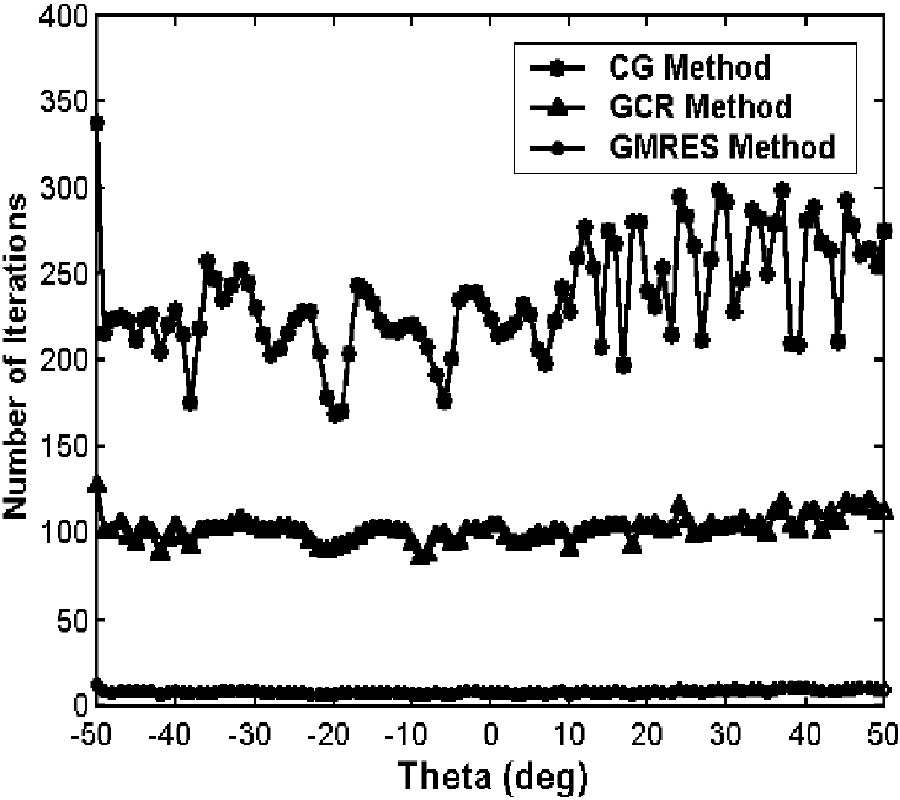PIER
Vol. 43, 101-121, 2003
Eigenfunctional Expansion of Dyadic Green's Functions in Gyrotropic Media Using Cylindrical Vector Wave Functions
This paper presents a novel eigenfunction expansion of the electric-type dyadic Green's function for an unbounded gyrotropic medium in terms of the cylindrical vector wave functions. The unbounded Green dyadics are formulated based on the Ohm-Rayleigh method, orthogonalityof the vector wave functions, and the newly formulated curl and divergence of dyadic identities. The irrotational part of the Green's function is obtained from the residual theorem. Unlike some of the published work where some assumptions are made prior to the formulation, the irrotational dyadic Green's function in this paper is formulated rigorouslybased on the idea given by Tai.
PIER
Vol. 43, 75-100, 2003
A Numerical Study of TM-Type Surface Waves on a Grounded Dielectric Slab Covered by a Doubly Periodic Array of Metallic Patches
In this paper we numerically study the propagation of surface waves guided by a metal-backed dielectric slab with biperiodic metallizations on its surface. Such structures are electromagnetic absorbing screens when the dielectric slab is lossy. In this paper, the surface waves are characterizedb y their longitudinal andtransv erse wave numbers, which are deduced from the complex pole locations of the reflection coefficient of the screens. The reflection coefficients can be obtainedwith a moment method. These reflection coefficients are generalizedto complex incident wave numbers. The poles are isolated in the complex plane with the help of the argument principle and are calculatedwith a numerical methodbasedon MÂ¨uller's algorithm. Then the parametric study of the wave numbers of the surface waves shows that the absorption of an electromagnetic wave by the screens at normal incidence is due to a resonance of the real part of the transverse wave number of the excitedsurface wave. We also show that there exists a Brewster incidence angle for the absorbing screens with suitable metallization array dimensions. This Brewster angle appears when the pole crosses the branch cut of the two-sheetedRiemann space of the reflection coefficient.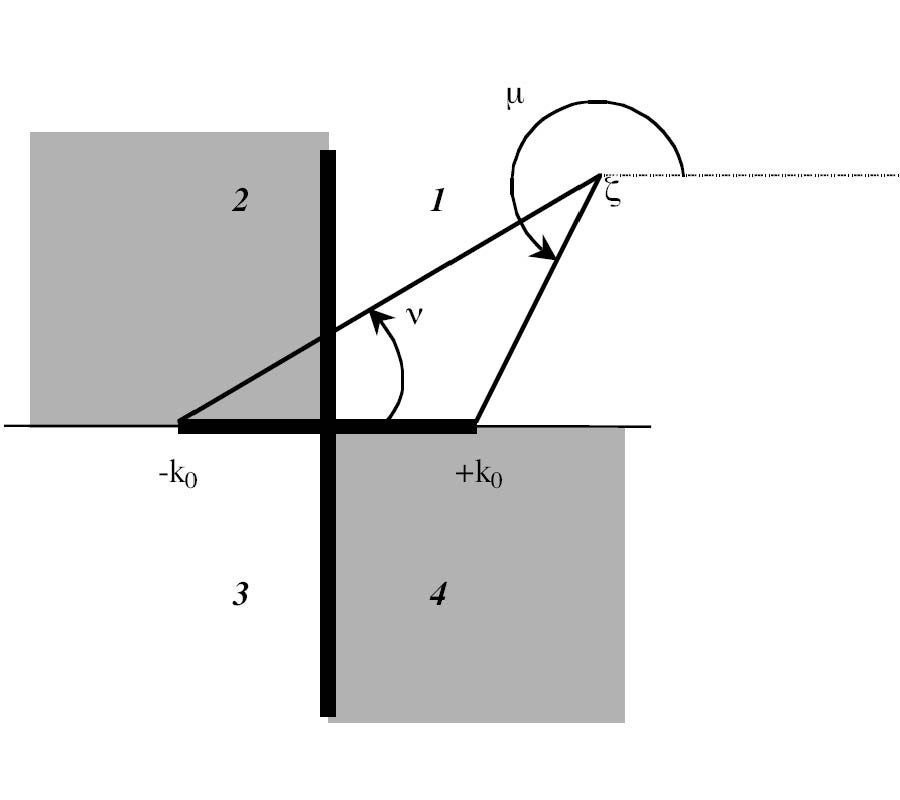PIER
Vol. 43, 59-74, 2003
Energy Patterns for a Conducting Circular Disc Buried in a Homogeneous Lossy Medium and Excited by Ultra-Wideband Generalized Gaussian Pulses
In this paper, we analyze the scattering of ultra-wideband (UWB) electromagnetic pulses from a conducting circular disc, buried in a homogeneous lossy medium. The transient currents excited on the surface of the conducting disc are derived, in the frequency domain, as series expansion of a set of orthogonal functions that satisfy specified boundary conditions. The amplitude spectral density of the surface currents are plotted for a given disc radius, and depth in a lossy medium. Aclosed form solution for the backscattered electric field strength in the far zone is derived in the frequency domain for the case of a normally-incident plane wave having the time variation of a generalized Gaussian pulse (GGP). The time variation and the energy density spectrum of the GGP signal and that of the backscattered signal in the far zone are plotted too. Computer plots of the backscattered energy versus observation angle, depth, disc radius, altitude from surface of the lossy medium, and the electric properties of the medium, result in various energy patterns that are desirable for the design and performance analysis of UWB ground-penetration impulse radar.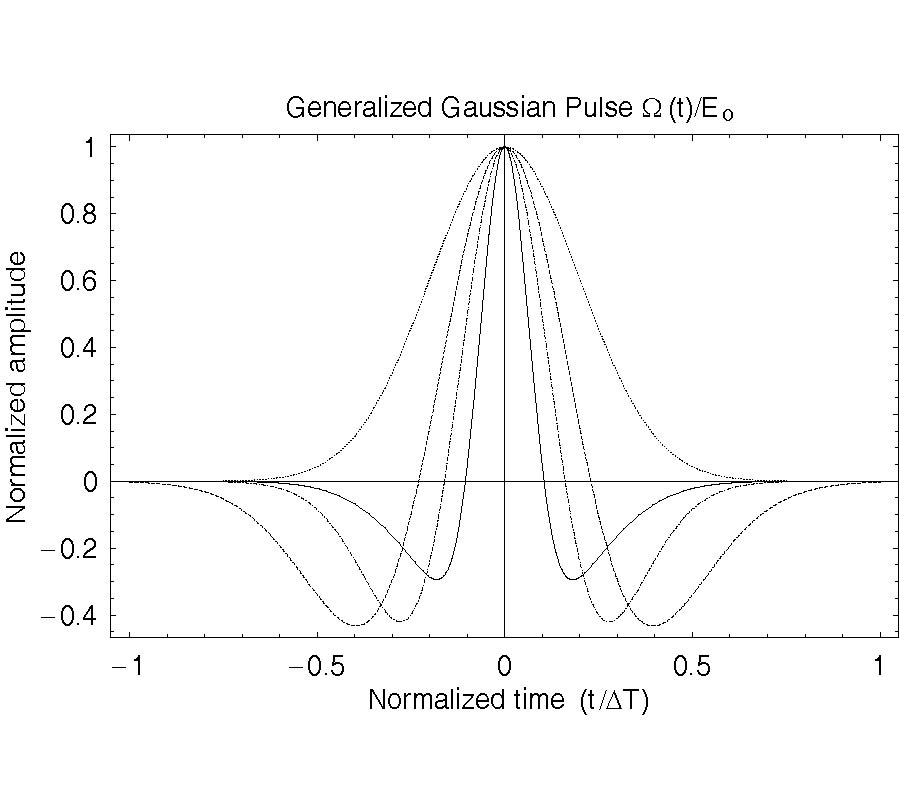PIER
Vol. 43, 39-58, 2003